Next: Taylor-Couette Flow Up: Incompressible Viscous Flow Previous: Flow Down an Inclined

# Poiseuille Flow

Steady viscous fluid flow driven by an effective pressure gradient established between the two ends of a long straight pipe of uniform circular cross-section is generally known as Poiseuille flow, because it was first studied experimentally by Jean Poiseuille (1797-1869) in 1838. Suppose that the pipe is of radius. Let us adopt cylindrical coordinates whose symmetry axis coincides with that of the pipe. Thus,measures distance along the pipe,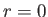corresponds to the center of the pipe, andcorresponds to the pipe wall. Suppose that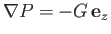(10.23)

is the uniform effective pressure gradient along the pipe, and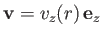(10.24)

the time independent velocity profile driven by this gradient. It follows from Section 1.19 that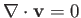and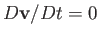. Hence, Equation (10.2) reduces to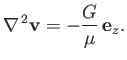(10.25)

Taking the-component of this equation, we obtain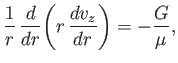(10.26)

where use has been made of Equation (1.155). The most general solution of the previous equation is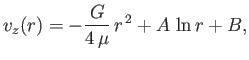(10.27)

whereand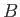are arbitrary constants. The physical constraints are that the flow velocity is non-singular at the center of the pipe (which implies that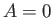), and is zero at the edge of the pipe [i.e.,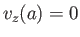], in accordance with the no slip condition. Thus, we obtain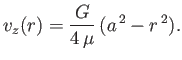(10.28)

The volume flux of fluid down the pipe is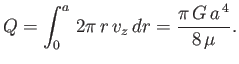(10.29)

According to the previous analysis, the quantity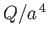should be directly proportional to the effective pressure gradient along the pipe. The accuracy with which experimental observations show that this is indeed the case (at relatively low Reynolds number) is strong evidence in favor of the assumptions that there is no slip at the pipe walls, and that the flow is non-turbulent. In fact, the result (10.29), which is known as Poiseuille's law, is valid experimentally provided the Reynolds number of the flow,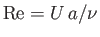, remains less than about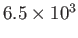. Here,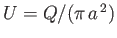is the mean flow speed. On the other hand, if the Reynolds number exceeds the critical valuethen the flow in the pipe becomes turbulent, and Poiseuille's law breaks down.Next: Taylor-Couette Flow Up: Incompressible Viscous Flow Previous: Flow Down an Inclined
Richard Fitzpatrick 2016-01-22